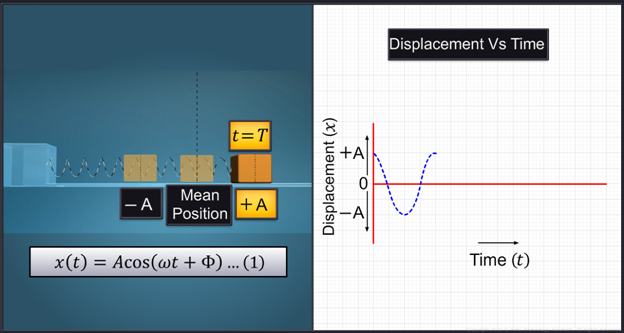Notes On Simple Harmonic Motion - CBSE Class 11 Physics
Consider a body attached to a spring. When the spring is compressed and released the body attached to it oscillates back and forth between the two extreme positions marked as plus A and minus A. As the body moves away from its mean position, it experiences a restoring force, which attempts to bring it back to the mean position.   This to-and-fro motion being executed by the body is called simple harmonic motion. The displacement of the particle from the origin is a function of time t.The displacement ‘x’ of a particle in simple harmonic motion can be expressed as x of t is   Written as the followingIn simple harmonic motion, the variation of the displacement of a particle with time t can be denoted by the displacement versus time graph.       A periodic motion where the displacement of an oscillating particle varies sinusoidally with time t can be called simple harmonic motion.   Since the displacement ‘x’ of the particle is dependent on the cosine function as given by the above equation the displacement will vary from +A to - A.   If time period of the particle in simple harmonic motion is T then at time t is equal to zero, the particle will be at the extreme position since x as expressed by Equation 1 will be equal to + A.   When time t is equal to T/4, the particle will be at mean position.   For the oscillating particle, when t=T/2, it is at the left extreme and when it is at t = 3T/4, it is again at the mean position.   When t = T, it returns to the right extreme position, plus A.   Amplitude of the motion:   The maximum displacement of a particle from the mean position executing simple harmonic motion is called its amplitude ‘A’.   In simple harmonic motion, the quantity, which varies with time is called the phase of the motion of the particle executing simple harmonic motion. At time t is equal to zero, the phase is equal toand this is called the phase constant or the phase angle. It is the initial phase of the particle executing simple harmonic motion.     In simple harmonic motion, amplitude is the only quantity that is time independent whereas displacement, velocity, acceleration and the phase angle, are time dependent.As shown in the graph, two simple harmonic motions may differ in the phase constant π in spite of having the samevalues.   For simplicity, if phase constant π is considered as zero, above equation (1) can be written asWe saw that when time‘t’ becomes equal to the time period, T, the displacement x becomes equal to A.   This implies thatWhereis called the angular frequency of the simple harmonic motion and its SI unit is radian per second.

#### Summary

Consider a body attached to a spring. When the spring is compressed and released the body attached to it oscillates back and forth between the two extreme positions marked as plus A and minus A. As the body moves away from its mean position, it experiences a restoring force, which attempts to bring it back to the mean position.   This to-and-fro motion being executed by the body is called simple harmonic motion. The displacement of the particle from the origin is a function of time t.The displacement ‘x’ of a particle in simple harmonic motion can be expressed as x of t is   Written as the followingIn simple harmonic motion, the variation of the displacement of a particle with time t can be denoted by the displacement versus time graph.       A periodic motion where the displacement of an oscillating particle varies sinusoidally with time t can be called simple harmonic motion.   Since the displacement ‘x’ of the particle is dependent on the cosine function as given by the above equation the displacement will vary from +A to - A.   If time period of the particle in simple harmonic motion is T then at time t is equal to zero, the particle will be at the extreme position since x as expressed by Equation 1 will be equal to + A.   When time t is equal to T/4, the particle will be at mean position.   For the oscillating particle, when t=T/2, it is at the left extreme and when it is at t = 3T/4, it is again at the mean position.   When t = T, it returns to the right extreme position, plus A.   Amplitude of the motion:   The maximum displacement of a particle from the mean position executing simple harmonic motion is called its amplitude ‘A’.   In simple harmonic motion, the quantity, which varies with time is called the phase of the motion of the particle executing simple harmonic motion. At time t is equal to zero, the phase is equal toand this is called the phase constant or the phase angle. It is the initial phase of the particle executing simple harmonic motion.     In simple harmonic motion, amplitude is the only quantity that is time independent whereas displacement, velocity, acceleration and the phase angle, are time dependent.As shown in the graph, two simple harmonic motions may differ in the phase constant π in spite of having the samevalues.   For simplicity, if phase constant π is considered as zero, above equation (1) can be written asWe saw that when time‘t’ becomes equal to the time period, T, the displacement x becomes equal to A.   This implies thatWhereis called the angular frequency of the simple harmonic motion and its SI unit is radian per second.

Next